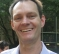## Power mapping from analogue domain(dBm) to digital domainStarted by 3 years ago7 replieslatest reply 3 years ago656 views

Hi All,

A standard says “-152dBm RF power should map to 1 LSB digital domain (at final stage).

In our case by the digital domain I mean the following stages: ADC (14 bits) => scaled up to 16 bits => apply fft with 0dB power gain (input to output with respect to 2K samples), select 1200 samples (18 bits) out of 2k samples (almost all power in them).

The ADC bitwidth, scaling, fft gain etc are user cases and could vary but the -152dBm mapping rule should be respected. If not then the power offset should be communicated.

I am not clear as how do I find out what is my offset.

Any views appreciated.

[ - ]You'd have to compute the LSB of the user selected gains and compare that to the -152dBm level.  Your offset will be the the distance from the LSB of the user to an LSB of -152dBm.  So if your settings get to -100dBm at LSB, the offset is 52 dBm.  That's how I would interpret it anyway.  Not sure that's what it means!

[ - ]Thanks Dr Mike,

I plan this method for an actual system case:

-70dBm RF gives -34dBFs after ADC.

fft maintains 0dB power transfer.

But I can't use dBFs across fft as 16bits map to 18bits and I can't use mean power as only 1200 bins out of 2k are chosen. The best parameter here is sum of power.

In fact that sum turned out to be 746268946 at input or output of fft.

I convert this sum to bits as log2(sqrt(746268946)) ~= 14.7

I convert bits to dB as 14.7*6 = 88.2dB

finally I mapped -70dBm to (-88.2dB -70dBm)  = -158.2 dB per bit

so in short what matters is that sum of power I get at the end irrespective of any other scaling. I assume this final figure will hold for any RF input power for a given system.

[ - ]I'm a little confused now - is the -70dBm the maximum input limit?  Then it makes sense because you have 88.2 dB range on a -70 dB peak.

I don't think you mean "-158.2 dB per bit", I think you mean -158.2 dB at LSB.  Because each bit is 6 dB more than the previous one.

-160 dB is mind bending to me since that's 10 picoWatts.  I bet my hand puts out more power than that at 10 cm, and I can't imagine how to deal with the device!

[ - ]-70 dBm is the actual RF mean power of given signal.

Yes the -152dBm mapping per bit is meant per lsb bit, a theoretical ratio and not meant to be physical.

I just tried more cases of our system with RF input mean power set to:

-42dBm, -47.2dBm,-50dBm, -60dBm,-80dBm & -100dBm

and following my plan I got mapping of about -158dBm per lsb bit after fft in all cases. So at least it is stable figures but still not sure about my interpretation.

[ - ]I would bet the spec means -152dBm RF input produces 1LSB rms output.  Since this level would be hard to measure due to quantization noise, it would be easier and more accurate to measure a higher power level and calculate (linearly) the level at 1LSB.  eg, inject -152 + 60dB = -92dBm and adjust digital gain to get (1+10)LSB rms.

Regarding DrMike's concern about the signal levels, these are perfectly normal and common RF levels.  The thermal noise floor is -174dBm/Hz, so -152dBm is reasonable for a noise floor (and probably why they spec that as the LSB).  A 2dB Noise Figure in a 100Hz Bandwidth would produce -152dBm at the output.

[ - ]Thanks Gordono, yes that is what I see as well by mapping -152 dBm RF to 1 lsb rms digital power. The minimum actual RF in our systems is -105 dBm.

What I say is that by just mapping dBm to dBFs at ADC then following gain in dBFs across processing modules to work out final rms is not doable and misleading. But instead I see using final sum of power and converting it to 1 lsb rms looks more sensible, here is my conversion of sum to lsb:

sum_pwr = sum(abs(x).^2); % x is the signal at final stage or equivalent

sum_pwr_bits = log2(sqrt(sum_pwr));

pwr_mapped_lsb  = rf_dbm - sum_pwr_bits*10*log10(2^2);

[ - ]